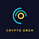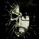1675 views
1675
Wavy Tunnel Trading Method
```study(title="Bungee", shorttitle="Bungee")

OverBought = hline(80)
OverSold = hline(20)

length1 = input(76, minval=1), smoothK1 = input(4, minval=1), smoothD1 = input(4, minval=1)
k1 = sma(stoch(close, high, low, length1), smoothK1)
plot(k1, color=orange)

length2 = input(3, minval=1), smoothK2 = input(3, minval=1), smoothD2 = input(3, minval=1)
k2 = sma(stoch(close, high, low, length2), smoothK2)
plot(k2, color=green)

length3 = input(16, minval=1), smoothK3 = input(3, minval=1), smoothD3 = input(3, minval=1)
k3 = sma(stoch(close, high, low, length3), smoothK3)
plot(k3, color=blue)

length4 = input(8, minval=1), smoothK4 = input(3, minval=1), smoothD4 = input(3, minval=1)
k4 = sma(stoch(close, high, low, length2), smoothK4)
plot(k4, color=red)

length5 = input(14, minval=1)
upper = highest(length5)
lower = lowest(length5)
WP = 100 * (upper - close) / (upper - lower)
plot(WP, color=purple)

length6 = input(2, minval=1)
up = rma(max(change(close), 0), length6)
down = rma(-min(change(close), 0), length6)
RSI = down == 0 ? 100 : up == 0 ? 0 : 100 - (100 / (1 + up / down))
plot(RSI, color=black)```Here you make mistake.

length5 = input(6, minval=2)
upper = highest(length5)
lower = lowest(length5)
WP = 100 + (-100 * (upper - close) / (upper - lower))
plot(WP, color=purple)